# A scale-based forward-and-backward diffusion process for adaptive image enhancement and denoising

## Abstract

This work presents a scale-based forward-and-backward diffusion (SFABD) scheme. The main idea of this scheme is to perform local adaptive diffusion using local scale information. To this end, we propose a diffusivity function based on the Minimum Reliable Scale (MRS) of Elder and Zucker (IEEE Trans. Pattern Anal. Mach. Intell. 20(7), 699-716, 1998) to detect the details of local structures. The magnitude of the diffusion coefficient at each pixel is determined by taking into account the local property of the image through the scales. A scale-based variable weight is incorporated into the diffusivity function for balancing the forward and backward diffusion. Furthermore, as numerical scheme, we propose a modification of the Perona-Malik scheme (IEEE Trans. Pattern Anal. Mach. Intell. 12(7), 629-639, 1990) by incorporating edge orientations. The article describes the main principles of our method and illustrates image enhancement results on a set of standard images as well as simulated medical images, together with qualitative and quantitative comparisons with a variety of anisotropic diffusion schemes.

## 1. Introduction

Different attributes such as noise, due to image acquisition, quantization, compression and transmission, blur or artefacts can influence the perceived quality of digital images , and requires post-processing such as image smoothing and sharpening steps for further image analysis including image segmentation, feature extraction, classification and recognition. In order to reduce noise while preserving spatial resolution, recent approaches use an adaptive approach by applying a combination of smoothing and enhancing filter to the image: image areas with little edges or sharpness are selectively smoothed while sharper image areas are instead processed with edge enhancing filters. Thus, the optimal strategy for noisy image enhancement is the combination of smoothing and sharpening that is adaptive to local structure of the image  with the aim of improving signal-to-noise ratio (SNR) and contrast-to-noise ratio (CNR)  of the image.

Scale-space methods in image processing have proven to be fundamental tools for image diffusion and enhancement. The scale-space concept was first presented by Iijima  and became popular later on by the works of Witkin  and Koenderink . The theory of linear scale-space supports edge detection and localization, while suppressing noise by tracking features across multiple scales . In fact, the linear scale-space is equivalent to a linear heat diffusion equation [13, 14]. However, this equation was found to be problematic as edge features are smeared and distorted after a few iterations. In order to overcome this problem, Perona and Malik  proposed an anisotropic diffusion partial differential equation (PDE), with a spatially constant diffusion coefficient. In their work, the term "anisotropic" refers to the case where the diffusivity is a scalar function varying with the location, it limits the smoothing of an image near pixels with a large gradient magnitude, which are essentially the edge pixels. Perona and Malik's work paved the way for a variety of anisotropic diffusive filtering methods , which have attempted to overcome the drawbacks and limitations of the original model and has produced significant advances. The main motivation for anisotropic diffusion is to reduce noise while minimizing image blurring across boundaries, but this process has several drawbacks, among them the disappearance of fine structures in low SNR or CNR regions and increased blurring in fuzzy boundaries. This is mainly due to the fact that the image gradient magnitude, on which the diffusion coefficient is estimated, is noisy and makes it difficult to distinguish between significant features and noise due to overlocalization, hence deciding edginess using the diffusion coefficient could be unreliable. In addition, traditional nonlinear diffusion filtering process does not offer any image-dependent guidance for selecting the optimum gradient magnitude at which the diffusion flow must have a maximum value . Moreover, as it was expressed by Black et al. , the choice of the diffusion coefficients could greatly affect the level of preservation of the edges.

In this article, based on early works on forward-and-backward (FAB) diffusion schemes [38, 50], where the smoothing and sharpening actions are combined in the same diffusion process system, we propose a scale-based forward-and-backward diffusion (SFABD) scheme. The main idea of the proposed scheme is that the magnitude of the diffusion coefficient at each pixel is determined by taking into account the local property of the image through the scales. This is performed using the notion of the Minimum Reliable Scale (MRS) as proposed by Elder and Zucker . This technique is based on statistical reliability of the edge detection operator responses at different scales . The reliable scale as defined by Elder and Zucker, means that at the selected scale and larger ones, the likelihood of error due to sensor noise is below a standard tolerance. By choosing the MRS, for edge detection at each pixel in the image, we prevent errors due to sensor noise while simultaneously minimizing errors due to interference from nearby structure. Such a multiscale measure along with the gradient can capture more accurately edges over a wide range of blur and contrasts. Using this concept, a MRS-based diffusivity function is proposed. As a result, the proposed scheme can adaptively encourage strong smoothing in homogeneous regions, while suitable sharpening in detail and edge regions. Furthermore, we modify the Perona-Malik  discrete scheme by taking edge orientations into account.

The remainder of this article is organized as follows: Section 2 gives an overview of the state-of-the-art anisotropic diffusion filtering; Sect. 3 presents the proposed SFABD algorithm; In Sect. 4, we illustrate image enhancement results on a set of well known test images as well as artificial medical images, and perform a qualitative and quantitative comparison of our method with a variety of anisotropic diffusion schemes. Finally, Sect. 5 states our concluding remarks.

## 2. Recent work on anisotropic diffusion

Perona and Malik  formulated anisotropic diffusion filtering as a process that encourages intraregional smoothing, while inhibiting interregional denoising. The Perona-Malik (P-M) nonlinear diffusion equation is of the form: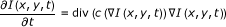(1)

where is the gradient operator, div is the divergence operator and c(·) is the diffusion coefficient, which is a non-negative monotonically decreasing function of local spatial gradient. If c(·) is constant, then isotropic diffusion is enacted. In this case, all locations in the image, including the edges, are equally smoothed. This is an undesirable effect because the process cannot maintain the natural boundaries of objects. The P-M discrete version of Equation 1 is given by: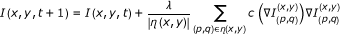(2)

where (x, y) denotes the coordinates of a pixel to be smoothed in the 2-D image domain, t denotes the discrete time step (iterations). The constant λ is a scalar that determines the rate of diffusion, η(x, y) represents the neighbourhood of pixel (x, y) and |η(x, y)| is the number of neighbours of pixel (x, y).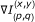indicates the image intensity difference between two pixels at (x, y) and (p, q) to approximate the image gradient. For the 4-connected neighbourhood's case, the directional gradients are calculated in the following way: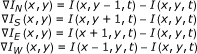(3)

In Perona-Malik's work , the diffusivity function has been defined as: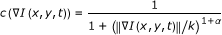, where α > 0

or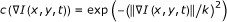(4)

where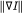is the gradient magnitude and the parameter k serves as a gradient threshold: a smaller gradient is diffused and positions with larger gradient are treated as edges. The P-M equation has several practical and theoretical drawbacks. As mentioned by Alvarez et al. , the continuous P-M equation is ill posed with the diffusion coefficients in (4); very close pictures can produce divergent solutions and therefore very different edges. This is caused by the fact that the diffusion coefficient c used in  leads to flux decreasing for some gradient magnitudes and the scheme may work locally as the inverse diffusion that is known to be ill posed, and can develop singularities of any order in arbitrarily small time. As a result, a large gradient magnitude no longer represents true edges and the diffusion coefficients are not reliable, resulting in unsatisfactory enhancement performance.

So far, much research has been devoted for improving the Perona-Malik's anisotropic diffusion method. For example, Catte et al.  showed that the P-M equation can be made well posed by smoothing isotropically the image with a scaling parameter σ, before computing the image gradient used by the diffusion coefficient: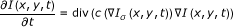(5)

Equation 5 corresponds to the regularized version of the P-M PDE, and I σ = G σ (I)*I is a smoothed version of I obtained by convolving the image with a zero-mean Gaussian kernel G σ of variance σ2. Similarly, Torkamani-Azar et al.  replaced the Gaussian filter with a symmetric exponential filter and the diffusion coefficient is calculated from the convolved image. Although these improvements can convert the ill-posed problem  in the Perona-Malik's anisotropic diffusion method into a well-posed one, their reported enhancement and denoising performance has been further improved. Weickert  proposed later a nonlinear diffusion coefficient that produces segmentation-like results given by: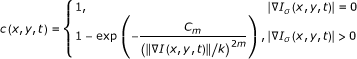(6)

where segmentation-like results are obtained using m = 4 and C4 = 3.31488.

Black et al.  proposed a more robust "edge-stopping" function derived from Tukey's biweight: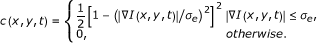(7)

where σ e is the "robust scale". Anisotropic smoothing with such edge stopping function can preserve sharper boundaries than previous schemes. Another diffusivity function, based on sigmoid function, has been proposed by Monteil and Beghdadi :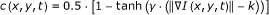(8)

where γ controls the steepness of the min-max transition region of anisotropic diffusion, and k controls the extent of the diffusion region in terms of gradient gray-level.

Notice that all of anisotropic diffusion filters presented above, utilize a scalar-valued diffusion coefficient (diffusivity function) c that is adapted to the underlying image structure, Weickert [26, 30, 55] defined this "pseudoanisotropy" as "isotropic nonlinear", and pointed out that the consequence of isotropic nonlinear diffusion is that only the magnitude, but not the direction of the diffusion flux can be controlled at each image location. Noise close to edge features remains unfiltered due to the small flux in the vicinity of edges. To enable smoothing parallel to edges, Weickert  proposed edge enhancing diffusion by constructing the diffusion tensor based on an orientation estimate obtained from observing the image at a larger scale: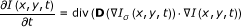(9)

where D is a 2 × 2 diffusion tensor and is specially designed from the spectral elements of the structure tensor to anisotropically smooth the image, while taking into account its intrinsic local geometry, preserving its global discontinuities.

For simultaneously enhance, sharpen and denoise images, Gilboa et al.  proposed a FAB adaptive diffusion process, denoted here as GSZFABD, where a negative diffusion coefficient is incorporated into image-sharpening and enhancement processes to deblur and enhance the extremes of the initial signal: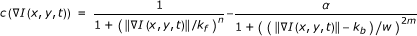(10)

where: k f has similar role as the k parameter in the P-M diffusion equation; k b and w define the range of backward diffusion, and are determined by the value of the gradient that is emphasized; α controls the ratio between the forward and backward diffusion; and the exponent parameters (n, m) are chosen as (n = 4, m = 1). Equation 10 is locally adjusted according to image features, such as edges, textures and moments. The GSZFABD diffusion process can therefore enhance features while locally denoising the smoother segments of images. Following the same ideas, and in order to avoid that the transition length between the maximum and minimum coefficient values varies with the gradient threshold, which makes controlling diffusion difficult, we proposed in  the local variance controlled forward-and-backward diffusion (LVCFABD) coefficient: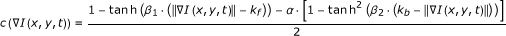(11)

where β1 and β2 control the steepness for the min-max transition region of forward diffusion and backward diffusion, respectively. These two parameters are vital to the FAB diffusion behaviour and the transition width from isotropic to oriented flux can be altered by modulating them. In addition, the obtained diffusion process can preserve the transition length from isotropic to oriented flux, and thus it is better at controlling the diffusion behaviour than the FAB diffusion of Gilboa et al. .

## 3. Scale-based forward-and-backward diffusion scheme

In this article, we propose a SFABD scheme combining the forward-backward scheme given by Equation 10 and the notion of MRS as proposed by Elder and Zucker . The MRS allows defining a classification map R(x, y), where each pixel (x, y) is classified into homogenous, edge or detail pixel. R(x, y) is then used in the coefficient α of Equation 10 to locally adapt the anisotropic diffusion. Finally, for implementing the SFABD scheme, we propose a modification of the P-M scheme by taking edge orientation into account.

### 3.1 Local scale-based classification map

In anisotropic diffusion scheme the rate of diffusion at each pixel is determined by diffusion coefficients that are monotonically decreasing functions of the gradient, thereby mainly ensuring strong smoothing in flat areas and weak diffusing near edge features. Thus, the strategy of identifying homogeneous and edge regions is very significant. Gradient is widely used to detect variable boundary in image processing, however, it is difficult for this measure to distinguish significant discontinuities from noise due to overlocalization. In addition, during anisotropic diffusion process, fine structures often disappear and increasing blurring occurs in fuzzy boundaries. To overcome this problem, we follow the idea of Elder and Zucker  of multiscale approach for edge detection, and explore the selection of proper scales for local estimation that depends upon the local structure of edges. The estimated scale is then used as a critical value and represents the MRS for each pixel in an image. The MRS proposed by Elder and Zucker  is based on two assumptions: (1) the noise comes from a stationary, zero-mean white noise process; (2) the standard deviation of the noise can be estimated from the image itself or by calibration. For the sake of clarity, the MRS is briefly described.

In edge detection, it is very important to estimate the nonzero gradient at each pixel in an image. The gradient computation from discrete data is an ill-posed problem. Smoothing the data with a Gaussian filter is the well-known regularization method. Hence, the gradient can be estimated using steerable Gaussian first derivative basis filters: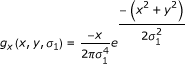(12)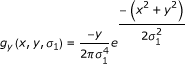(13)

where σ1 denotes the scale of the first derivative Gaussian kernel g(x, y, σ1). The magnitude and direction of the gradient in an image I(x, y) are given by: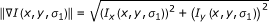(14)

where: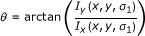(15)

θ is the gradient vector direction at (x, y). I x (x, y, σ1) and I y (x, y, σ1) are defined as: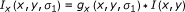(16)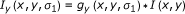(17)

In gradient-based edge detection, the local gradients in a homogeneous region due to noise will have a nonzero value. Thus, the likelihood that the response of the gradient operator induced by noise should be respected. Considering the derivative operation as a random process transformation, the probability distribution function (PDF) of a noise gradient can be represented as [56, 57]: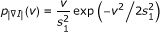(18)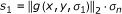(19)

where the L2 norm of the first derivative operator is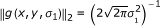, σ n is the standard deviation of the sensor noise, and σ1 is the scale of the Gaussian kernel. Elder and Zucker  defined the MRS as the unique scale at which the events can be reliably detected. By reliable, they mean that at this and larger scales, the likelihood of error due to sensor noise is equal to or below a predetermined false positive rate. Reliability is defined in terms of a Type I (false positive) error, α I , for the entire image and a point-wise Type I error, α p . Under the assumption of i.i.d. noise, the point-wise Type I error α p can be computed from the probability of having no false positive edges as follows :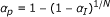(20)

where N is the total number of pixels in the image. By selecting the MRS, the error due to sensor noise is limited while the interference of neighbourhood structures is minimized. Given the probability distribution function (pdf) of gradient of the noise in equation (18), point-wise Type I error α p is defined when using a gradient threshold c1 to detect an edge: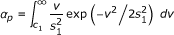(21)

Using the above equation, and considering a fixed type I error, we can define a critical value function: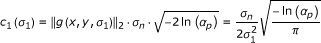(22)

Giving a point-wise Type I error α p , c1(σ1) represents the statistically reliable minimum gradient response based on the sensor noise and operator scale. Figure 1 depicts the critical value function c1(σ1) for different noise levels and different Type I error rates. It is easy to observe that c1(·) is a non-negative monotonically decreasing function of σ1, which is helpful in detecting blurred boundaries. Comparing Figure 1a and 1b, we notice that c1(σ1) is more sensitive to the standard deviation of sensor noise σ n than to the Type I error α I . Furthermore, c1(σ1) grows as σ n increases, for eliminating spurious edges in the presence of highly corrupted images. In this article, a thin-plate smoothing spline model is used to smooth a given image. It is assumed that the model whose generalized cross-validation score is minimum can provide the variance of the sensor noise σ n .

For the MRS algorithm, how to sample the scale space is an open question. In scale space theory and for natural images, it is known that logarithmic scale is sufficient to represent the scale space completely . For example, Elder and Zucker  used six logarithmic scales σ1 = {0.5, 1, 2, 4, 8, 16} in their experiments. Table 1 summarises the alternative sampling schemes for scale space, both the Logarithmic and Limited-Log methods are logarithmic scales, while the latter has a shorter coverage. The Linear method samples the scale uniformly, and the Linear-Log one is a combination of Linear and Logarithmic. In this work, we empirically found the following linear sampling gives good results: σ1 = {0.6, 0.9, 1.2, 1.5,..., 2.4}. In our implementation, we select the MRS at each pixel as the smallest scale at which the gradient estimate exceeds the critical value function: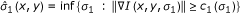(23)

Strictly speaking, if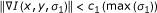, the pixel usually resides in homogeneous regions and the MRS can be defined as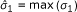, while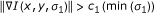, the pixel may correspond to edge or detailed feature and the MRS is chosen as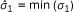.

Finally, we define the scale-based classification map R(x, y) as follows: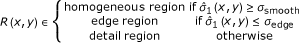(24)

where R(x, y) denotes the region type of pixel (x, y). It has to be noted that the proper modulation of the thresholds σsmooth and σedge is required for a robust classification map. As an example, the classification map of the Cameraman image and its noisy version (σ2 = 225) are illustrated in Figures 2b and 2d, respectively. In the map, black regions are homogeneous, gray regions represent detail regions, while white regions manifest inhomogeneities that indicate most of the important features, e.g., the camera and tripod. This example clearly illustrates that the scale-based classification map readily indicate locations of highly homogeneous, detail and edge regions.

### 3.2 Scale-based forward-and-backward diffusion coefficient

As shown in [59, 60], if the weight α in (10-11) is constant for all pixels in an image, this diffusion coefficient (10) is positive for small image gradients, while it becomes negative for large ones, and finally becomes positive again. Different nonlinear diffusion behaviours can be obtained by varying the value of α. For example, when α is large, the backward diffusion force is dominant. The larger α is, the more sharpening occurs. However, a too large α easily results in oscillations. Conversely, when α is small, the diffusion process performs image smoothing. Small values of α increase the noise attenuation at the price of a possible increase of detail blur. Thus, the optimal choice depends on the amount of noise variance and is typically a trade-off between smoothing and sharpening. Nevertheless, Gilboa et al.  proposed that the same α is used for the entire image, regardless of local structures of an image, which leads to over-smoothing in edge or detail regions and under-smoothing in homogeneous regions. In this article, we propose the balancing weight α, with different values δsmooth, δedge and δdetail , selectively applied at each pixel following the value of the local scale-based classification map R(x, y). Indeed, in the edge areas, the image requires more sharpening to highlight important features embedded in it, while in the detail regions, the diffusion process should compromise the effects of smoothing and sharpening, ensuring that the backward force can emphasize the fine structures in the image and the stabilizing forward force is strong enough to avoid oscillations. Whereas, in homogeneous regions, the gradient magnitude is very slow after the Gaussian pre-smoothing is applied to reduce the background noise. Thus, the approximate isotropic diffusion is performed to uniformly smooth the gentle and flat areas. In this way, each pixel is adaptively assigned a different parameter by evaluating the local structures. This is made possible using the MRS-based diffusivity function: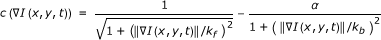(25)

with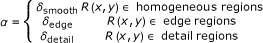(26)

where δsmooth, δedge and δdetail are the scale-based weights, selected empirically such that δedgeδdetailδsmooth ≥ 0. Kf and kb control the MRS-based diffusivity function for forward and backward diffusion, respectively. As mentioned above, the parameter kf has the same role as the gradient threshold in the P-M diffusion equation. Thus, the mean of local intensity differences in homogeneous regions is effective for controlling the forward diffusion; while kb is determined by the value of the gradient that is emphasized. Previous works [38, 59] demonstrated that kb is several times larger than kf, in our case, we empirically defined the two gradient thresholds in (25) as [kf, kb] = [1, 2]*k. This strategy is indeed required in cases of natural signals or images because of their nonstationary structure. Usually, a minimal value of forward diffusion should be kept, so that large smooth areas do not become noisy. For the estimation of k, we use the assumption of i.i.d. noise, indeed, since the noise is assumed to be randomly distributed in the image space, a practical way of estimating its variance is to consider homogeneous regions where small variations or textures are mainly due to noise. Thus, k is estimated as the mean of the local intensity differences on the homogeneity map, i.e.,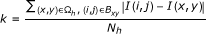(27)

where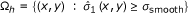and B xy is the neighbourhood set of pixel (x, y), and N h is the total number of pixels in the homogeneous regions as defined by the classification map R(x, y). When Ω h is empty, the simplest idea might be to setup k as a user defined constant, or using a "noise estimator": a histogram of the absolute values of the gradient throughout the image is computed, and k is set greater than or equal to e.g. 90% value of its integral at each iteration.

### 3.3 Edge orientation driven discretization scheme (EODDS)

As mentioned in Sect. 3.1, three different regions are classified before diffusion evolution. However, edge orientation is not taken care in the discrete scheme of P-M anisotropic diffusion. As a result, they are always considered to be displaced vertically or horizontally . Moreover, one cannot recognize whether a slight intensity variation is mainly due to a slow varying edge or noise, so it is unreasonable that both situations are treated in the same way. The anisotropic diffusion discrete scheme should be modified to take edge orientations into account in the detail and edge regions, i.e. filtering action should be rather stronger on the direction parallel to the edge, and weaker on the perpendicular direction. Hence, we discretized the original anisotropic diffusion equation as follows: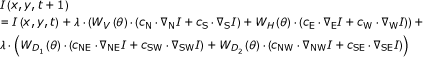(28)

where the mnemonic subscripts N, S, E, W, NE, SW, NW and SE denote the eight directions North, South, East, West, North-East, South-West, North-West and South-East, and the symbol stands for nearest-neighbour differences. λ is the time step for the numerical scheme; θ is the edge direction at pixel (x, y), WV(θ), WH(θ),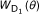and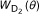are weights for different edge directions.

For a nonlinear diffusion scheme, stability is an important issue that concerns possible unbounded growth or boundness of the final result of the diffusion scheme. The essential criterion defining stability is that the numerical process must restrict the amplification of all components from the initial conditions. In the following, we describe how to find the maximum value of λ assuring the stability. Assuming N d the dimension of the neighborhood in direction d (in the vertical or horizontal direction for 4-connected neighbourhood, N d = 1), the stability condition is given by :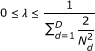where D is the dimension of a given image. For our case (2-D images and 8-connected neighborhood), the condition becomes: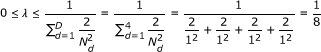In this article, the step of keypoint orientation in scale-invariant feature transform (or SIFT)  algorithm is used for estimating the edge direction. The image is subdivided into nonoverlapping blocks of the same size, typically between 8 × 8 and 32 × 32 pixels. The gradient-based edge orientation histogram is then calculated in each block. If we let N be the total number of pixels in the image and n be the total number of bins, the histogram H i meets the following conditions: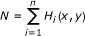(29)

In the histogram, 360 degree is grouped in 36 groups, each of which is π/18 degree, and we obtain n = 36. Thus, the main orientation in each block is defined as follows: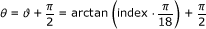(30)

and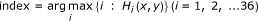(31)

where ϑ is the main gradient direction, by calculating the histogram of the gradient direction for each pixel (x, y) in the block, and "arctan" is the inverse tangent function. We assume that if an intensity variation between two zones is present, the edge has to be located along the perpendicular direction. The calculation of orientation histogram can be performed in real time. Furthermore, the comparison of orientation histograms can be performed using Euclidian distance that is very fast to compute for vectors whose dimensions are 36.

Once the estimation of the edge direction has been performed, the weights Wv(θ), WH(θ),andhave to be defined, in such a way that they satisfy the following constraint, with the aim of maintaining the numerical stability of the process: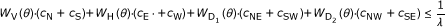(32)

In order to illustrate the way the weights are estimated, we divide the x - y plane into five domains as follows (see Figure 3):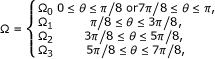(33)

Taking the constraint (32) and the trigonometric relation into account, the weights Wv(θ), WH(θ),andare estimated as: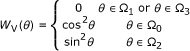(34)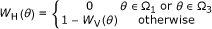(35)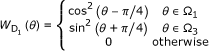(36)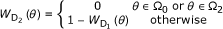(37)

For instance, if θ Ω0, substituting these weights in the modified anisotropic diffusion Equation 29 leads to the following: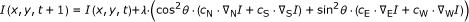(38)

In this case, the edge orientation should approximate the vertical direction according to the fact that the edge direction is always perpendicular to the gradient direction. During the diffusion process, a relatively large weight cos2θ is assigned in the vertical direction to guarantee that the diffusion should mainly occur in the direction parallel to the edge, while a relatively small weight sin2θ is assigned in the horizontal direction to ultimately avoid diffusion across the edge.

### 3.4 SFABD algorithm

The algorithm for the proposed SFABD scheme is summarised in Algorithm 1.

## 4. Experiments

Chen  classified the existing performance evaluation methods into three categories; i.e. subjective, objective and application-based methodologies. By the subjective methodology, a noisy image and its enhanced images are illustrated. Thus, the evaluation on the performance of an algorithm is dependent on human's common sense gained from very much sophisticated visual perception experience. By the objective methodology, an evaluation is performed by comparing the enhanced image and its original uncorrupted version to see how much noise has been removed from a noisy image. By the application-based methodology, images in a certain application field are used for test and the enhancing results are assessed by a specialist who has expertise in the field or a comparison with an anticipated result set up prior to the test.

To assess the proposed approach, we follow the above-described methodology and demonstrate the effectiveness of SFABD in enhancing fine edge structures, i.e. we applied it to a variety of blurred and noisy images by comparing its results to five counterparts, namely, the Catte's anisotropic diffusion (CAD) , the robust anisotropic diffusion (RAD) , the Monteil's anisotropic diffusion (MAD) , the Weickert's anisotropic diffusion (WAD) , and the edge-enhancing diffusion (EED) . The gradient threshold k should be chosen according to the noise level and the edge strength. In our experiments, we set k in different diffusion algorithms by referring to the original papers. The ultimate goal of image enhancement is to facilitate the subsequent processing for early vision. To demonstrate the usefulness of our algorithm in an early vision task, we apply our algorithm for performing edge-enhancing filtering on medical images, for an application-based evaluation.

In order to objectively evaluate the performance of the different diffusion algorithms, we adopt two noise-reduction measures: peak signal-to-noise ratio (PSNR) and the universal image quality index (UIQI). The measure of PSNR has been widely used in evaluating performance of a smoothing algorithm in the objective methodology. For a given noisy image I, I(i, j, T) denotes the intensity of pixel (x, y) I at iteration T while an anisotropic diffusion algorithm is applied to the noisy image. G(i, j) is its uncorrupted ground-truth. As a result, the PSNR is defined as follows:(39)

Here, MAX I is the maximum gray value of the image. When the pixels are represented using 8 bits per sample, MAX I = 225. Typical values for the PSNR in lossy image and video compression are between 30 and 50 dB, where higher is better. Acceptable values for wireless transmission quality loss are considered to be about 20 to 25 dB [64, 65]. Recently, the UIQI has been used to better evaluate image quality due to its strong ability in measuring structural distortion occurred during the image degradation processes :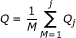(40)

where M is the total step number and Q j denotes the local quality index computed within a sliding window. In this article, a sliding window of size 8 × 8 is applied to estimate an entire image. The dynamic range of Q is [-1,1], the value 1 is only achieved if the compared images are identical and the value of -1 means lowest quality of the distorted image.

### 4.1 General images

The performance of the proposed algorithm is evaluated using four standard images of size 512 × 512 and 256 gray-scale values. The image of Peppers is employed as an example of piecewise-constant image. The Lena and Cameraman images are two examples with both textures and smooth regions. The Boat image is an example with different edge features. For performance evaluation, the images have been corrupted with additive Gaussian white noise with different noise levels. The PSNR and UIQI values of the four noisy images with respect to different noise variance are listed in Table 2. The Lena and Boat images and their noisy versions with noise variance 225 are displayed in Figures 4 and 5, respectively. For clarity, only selected regions of the images are displayed.

Second, the proposed SFABD algorithm has been also compared to three existing FAB diffusion schemes, namely the GSZFABD , LVCFABD  and tunable FAB diffusion (TFABD) , using visual quality and the PSNR and UIQI values. Figure 8 depicts the obtained results of the considered FAB diffusion schemes. One can notice that all the four FAB processes can achieve a good compromise between sharpening and denoising. However, as illustrated in Figure 8a, the GSZFABD process blurs edges and detail features. From Figures 8b, c, it can be seen that the LVCFABD and TFABD schemes are sensitive to noise: the LVCFABD results in developing singularities in homogeneous regions, such as the inner parts of peppers, while the TFABD causes oscillations in the vicinity of edges, e.g. the outer contour of peppers. However, the proposed SFABD scheme exhibits the best edge-enhancing diffusion behaviour. The quantitative results of the four schemes are given in Table 4. It is evident from Table 4 that the SFABD scheme is much more efficient than the other three schemes for the four images. Hence, we can say that SFABD outperforms the existing FAB enhancement techniques.

In order to appraise the effectiveness of the adaptive gradient threshold, the gradient threshold k f curves for four noisy images (σ2 = 400) are depicted in Figure 9. It can be seen that all the curves, representing the evolution of this parameter, share the same decreasing behaviour as already demonstrated in other works, allowing lesser and lesser gradients to take part in the diffusing process. Moreover, after 20 iterations, k f decreases slower and slower and the scheme converges to a steady state where for t → ∞, we get c(|I|) → 0, which means that almost no diffusion is performing. Note that, the estimation of an optimum threshold value k has been addressed by several authors [29, 50, 67, 68]. However, to our knowledge, these authors do not explain how to determine the homogenous regions during the process. In this work, an appropriate solution for automatically adapting the gradient threshold at each iteration has been proposed.

### 4.2 Medical images

In medical images, low SNR and CNR often degrade the information and affect several image processing tasks, such as segmentation, classification and registration. Therefore, it is of considerable interest to improve SNR and CNR to reduce the deterioration of image information. In this section, we report the results of the proposed SFABD scheme on two three-dimensional MR images [69, 70], both of which have been simulated using two sequences (T1- and T2-weighted) with 1 mm of slice thickness, 9% noise level and 20% of intensity non-uniformity downloaded from Brainweb  using default acquisition parameters for each modality. These simulations are based on an anatomical model of normal brain, which can serve as ground truth for any analysis procedure.

Figures 10 and 11 show two examples of enhanced MR image using different diffusion schemes. The original noise-free images and their corrupted versions are illustrated in Figures 10a, b and 11a, b, respectively. As expected, the six algorithms remove noise and simultaneously smooth the homogeneous regions, such as white matter. However, for RAD, noise is still remaining in the resulting images. Some structure details are not visible in the images restored by the CAD, WAD and MAD algorithms, though they can greatly attenuate the effect of noise. According to the visual analyses of the image quality, the results given by the EED diffusion and the proposed SFABD are comparable, because the two processes perform edge-enhancing diffusion. Nevertheless, the SFABD scheme achieves better contrast and produces more reliable edges, which is especially useful for segmentation and classification purposes necessary in medical image applications.

In order to objectively evaluate the performances of the different diffusion algorithms on medical images, we adopt the PSNR and the Structural Similarity (SSIM) measure . SSIM is a quality metric that measures the presence of the image structure details in the restored images and the value one is only achieved if the compared images are identical. The lowest value is zero if the images show no similarity at all. Since both the considered MR simulated images are three-dimensional data volume, we compare the PSNR and SSIM values at each slice for objective evaluation. As shown in Figure 12, the PSNR values of the restored images achieved by the proposed SFABD scheme are comparable or higher than the other diffusion algorithms, and the SSIM values of SFABD are significantly higher. Finally, the proposed scheme enhances boundary sharpness and fine structures better than other considered diffusion methods.

## 5. Conclusion

We have presented a novel SFABD scheme for image restoration and enhancement. In the proposed scheme, the magnitude of the diffusion coefficients at each pixel is determined by taking into account the property of the image through scale-space, using a classification map obtained via the MRS. According to the type of the considered pixel (belonging to a homogenous, detail or edge region), a variable weight is incorporated into the anisotropic diffusion PDE to adaptively encourage strong smoothing in homogeneous regions and suitable sharpening in detail and edge regions. Moreover, we propose a method to estimate the parameter k of MRS-based diffusivity function, as the mean of the local intensity differences on homogeneous regions as determined by the MRS-based classification map. Finally, a numerical scheme, taking into account the edge orientation has been proposed. Furthermore, extensive qualitative and quantitative comparisons with a variety of existing diffusion schemes demonstrate the effectiveness of the proposed scheme, along with its potential use for medical image applications. Future work will involve two main aspect of the proposed approach, namely an adaptive approach for the estimation of the parameters, as well as establishing an automatic stopping criterion to replace the prefixed numbers of iteration for anisotropic diffusion.

## Algorithm 1. Scale-based forward-and-backward diffusion

1. 1.

Initialize the image data I. I (x, y, 0) denotes the original intensity of pixel (x, y).

2. 2.

Initialize the diffusion parameters. Set the values of the of the noise scale σ, the maximum number of iterations T, the classification map thresholds σ smooth and σ edge, and the scale-based weights δ smooth, δ edge and δ detail.

3. 3.

Calculate the critical value for each pixel and determine its region type.

4. a.

Obtain the regularized image I σ .

5. b.

Compute the gradient of the smoothed image, I σ = (d x ,d y ) T .

6. c.

Calculate the critical value for each pixel.

7. d.

Determine the minimum reliable scale of each pixel by using the relationship between the spatial gradient and the critical value (23).

8. e.

Estimate the classification map R(x, y) in (24)

9. 4.

Iterate the diffusion filtering until t = T.

10. a.

The gradient thresholds k f and k b are estimated as discussed in Section 3.2.

11. b.

For each pixel (x, y), the diffusion coefficient c() is computed using Eq. (25). In homogeneous and detail regions, the traditional 4-connected neighbourhood diffusion discretization equation is performed to update I(x, y, t); while in edge regions, the 8-connected neighbourhood diffusion discretization equation (28) is performed to update I(x, y, t).

## Abbreviations

Catte's anisotropic diffusion

CNR:

contrast-to-noise ratio

EED:

edge-enhancing diffusion

EODDS:

edge orientation driven discretization scheme

FAB:

forward-and-backward

LVCFABD:

local variance controlled forward-and-backward diffusion

Monteil's anisotropic diffusion

MRS:

Minimum Reliable Scale

PDE:

partial differential equation

pdf:

probability distribution function

P-M:

Perona-Malik

PSNR:

peak signal-to-noise ratio

robust anisotropic diffusion

SIFT:

scale-invariant feature transform

SFABD:

scale-based forward-and-backward diffusion

SNR:

signal-to-noise ratio

SSIM:

Structural Similarity

TFABD:

tunable FAB diffusion

UIQI:

universal image quality index

Weickert's anisotropic diffusion.

## References

1. 1.

Damera-Venkata N, Kite TD, Geisler WS, Evans BL, Bovik AC: Image quality assessment based on a degradation model. IEEE Trans Image Process 2000,9(4):636-650. 10.1109/83.841940

2. 2.

Russo F: An image enhancement technique combining sharpening and noise reduction. IEEE Trans Instrum Meas 2002,51(4):824-828. 10.1109/TIM.2002.803394

3. 3.

Lee JS: Digital image enhancement and noise filtering by use of local statistics. IEEE Trans Pattern Anal Machine Intell 1980, PAMI-2: 165-168.

4. 4.

Chan P, Lim J: One-dimensional processing for adaptive image restoration. IEEE Trans Acoust Speech Signal Process 1985,33(1):117-126. 10.1109/TASSP.1985.1164534

5. 5.

Wang DCC, Vagnucci AH, Li CC: Gradient inverse weighted smoothing scheme and the evaluation of its performance. Comput Graphics Image Process 1981,15(2):167-181. 10.1016/0146-664X(81)90077-0

6. 6.

Rank K, Unbehauen R: An adaptive recursive 2-D filter for removal of Gaussian noise in images. IEEE Trans Image Process 1992,1(3):431-436. 10.1109/83.148617

7. 7.

Ahn CB, Song YC, Park DJ: Adaptive template filtering for signal-to-noise ratio enhancement in magnetic resonance imaging. IEEE Trans Med Imaging 1999,18(6):549-556. 10.1109/42.781019

8. 8.

Smith SM, Brady JM: SUSAN-A New Approach to Low Level Image Processing. Int J Comput Vision 1997,23(1):45-78. 10.1023/A:1007963824710

9. 9.

Iijima T: Basic theory of pattern observation. Papers of Technical Group on Automata and Automatic Control 1959.

10. 10.

Iijima T: Basic theory on normalization of a pattern (in case of typical one-dimensional pattern). Bull Electr Lab 1962, 26: 368-388.

11. 11.

Weickert J, Ishikawa S, Imiya A: Linear Scale-Space has First been Proposed in Japan. J Math Imaging Vision 1999,10(3):237-252. 10.1023/A:1008344623873

12. 12.

Witkin AP: Scale-space filtering. Proceedings of International Joint Conference on Artificial Intelligence, New York 1983, 1019-1021.

13. 13.

Koenderink JJ: The structure of images. Biol Cybern 1984, 50: 363-370. 10.1007/BF00336961

14. 14.

Koenderink JJ, Doorn AJV: Generic neighborhood operators. IEEE Trans Pattern Anal Machine Intell 1992,14(6):597-605. 10.1109/34.141551

15. 15.

Lindeberg T: Feature detection with automatic scale selection. Int J Comput Vision 1998,30(2):77-116.

16. 16.

Yuille AL, Poggio T: Scaling theorems for zero-crossings. IEEE Trans Pattern Anal Machine Intell 1986,8(1):15-25.

17. 17.

Babaud J, Witkin AP, Baudin M, Duda RO: Uniqueness of the Gaussian kernel for scale-space filtering. IEEE Trans Pattern Anal Machine Intell 1986,8(1):26-33.

18. 18.

Elder JH, Zucker SW: Local scale control for edge detection and blur estimation. IEEE Trans Pattern Anal Machine Intell 1998,20(7):699-716. 10.1109/34.689301

19. 19.

Catte F, Lions PL, Morel JM, Coll T: Image selective smoothing and edge detection by nonlinear diffusion. SIAM-JNA 1992,29(1):182-193.

20. 20.

Alvarez L, Lions PL, Morel JM: Image selective smoothing and edge detection by nonlinear diffusion. SIAM-JNA 1992,29(3):845-866.

21. 21.

Gerig G, Kubler O, Kikinis R, Jolesz FA: Nonlinear anisotropic filtering of MRI data. IEEE Trans Med Imaging 1992,11(2):221-232. 10.1109/42.141646

22. 22.

Nitzberg M, Shiota T: Nonlinear image filtering with edge and corner enhancement. IEEE Trans Pattern Anal Machine Intell 1992,14(8):826-833. 10.1109/34.149593

23. 23.

Whitaker RT, Pizer SM: A multi-scale approach to nonuniform diffusion. Comput Vision Graphics Image Process Image Underst 1993, 57: 99-110. 10.1006/cviu.1993.1006

24. 24.

Alvarez L, Mazorra L: Signal and Image Restoration Using Shock Filters and Anisotropic Diffusion. SIAM J Numer Anal 1994,31(2):590-605. 10.1137/0731032

25. 25.

Li X, Chen T: Nonlinear diffusion with multiple edginess thresholds. Pattern Recognit 1994,27(8):1029-1037. 10.1016/0031-3203(94)90142-2

26. 26.

Weickert J: Theoretical foundations of anisotropic diffusion in image processing. Computing 1996, 11: 221-236.

27. 27.

Fischl B: EL Schwartz, Learning an Integral Equation Approximation to Nonlinear Anisotropic Diffusion in Image Processing. IEEE Trans Pattern Anal Machine Intell 1997,19(4):342-352. 10.1109/34.588012

28. 28.

Acton ST: Multigrid anisotropic diffusion. IEEE Trans Image Process 1998,7(3):280-291. 10.1109/83.661178

29. 29.

Black MJ, Sapiro G, Marimont DH, Heeger D: Robust anisotropic diffusion. IEEE Trans Image Process 1998,7(3):421-432. 10.1109/83.661192

30. 30.

Weickert J: Anisotropic Diffusion in Image Processing. BG Teubner, Stuttgart; 1998.

31. 31.

Weickert J, Romeny BMTH, Viergever MA: Efficient and reliable schemes for nonlinear diffusion filtering. IEEE Trans Image Process 1998,7(3):398-410. 10.1109/83.661190

32. 32.

Fischl B, Schwartz EL: Adaptive nonlocal filtering: a fast alternative to anisotropic diffusion for image enhancement. IEEE Trans Pattern Anal Machine Intell 1999,21(1):42-48. 10.1109/34.745732

33. 33.

Monteil J, Beghdadi A: A new interpretation of the nonlinear anisotropic diffusion for image enhancement. IEEE Trans Pattern Anal Machine Intell 1999,21(9):940-946. 10.1109/34.790435

34. 34.

Acton ST: Locally monotonic diffusion. IEEE Trans Signal Process 2000,48(5):1379-1389. 10.1109/78.839984

35. 35.

Pollak I, Wilsky AS, Krim H: Image segmentation and edge enhancement with stabilized inverse diffusion equations. IEEE Trans Image Process 2000,9(2):256-266. 10.1109/83.821738

36. 36.

Sapiro G: Geometric Partial Differential Equations and Image Analysis. Cambridge University Press, Cambridge; 2001.

37. 37.

Aubert G, Kornprobst P: Mathematical Problems in Image Processing: Partial Differential Equations and the Calculus of Variations. Springer Verlag, New York; 2002.

38. 38.

Gilboa G, Sochen N, Zeevi YY: Forward-and-backward diffusion processes for adaptive image enhancement and denoising. IEEE Trans Image Process 2002,11(7):689-703. 10.1109/TIP.2002.800883

39. 39.

Mrázek P, Navara M: Selection of Optimal Stopping Time for Nonlinear Diffusion Filtering. Int J Comput Vision 2003,52(2/3):189-203. 10.1023/A:1022908225256

40. 40.

Gilboa G, Zeevi NSYY: Image Enhancement and Denoising by Complex Diffusion Processes. IEEE Trans Pattern Anal Machine Intell 2004,25(8):1020-1036.

41. 41.

Young-Chul S, Doo-Hyun C: Scale-based image enhancement using modified anisotropic diffusion filter. Opt Eng 2004,43(9):2094-2099. 10.1117/1.1778730

42. 42.

Tschumperle D, Deriche R: Vector-valued Image Regularization with PDEs: A Common Framework for Different Applications. IEEE Trans Pattern Anal Machine Intell 2005,27(4):1-12.

43. 43.

Duarte-Carvajalino JM, Castillo PE, Velez-Reyes M: Comparative Study of Semi-Implicit Schemes for Nonlinear Diffusion in Hyperspectral Imagery. IEEE Trans Image Process 2007,16(5):1303-1314.

44. 44.

Wang Y, Zhang L, Li P: Local Varianced-Controlled Forward-and-Backward Diffusion for Image Enhancement and Noise Reduction. IEEE Trans Image Process 2007,16(7):1854-1864.

45. 45.

Zhong J, Sun H: Wavelet-Based Multiscale Anisotropic Diffusion With Adaptive Statistical Analysis for Image Restoration. IEEE Trans Circuits Syst 2008,55(9):2716-2725.

46. 46.

Wang Y, Niu R, Yu X: Anisotropic Diffusion for Hyperspectral Imagery Enhancement. IEEE Sensors J 2010,10(3):469-477.

47. 47.

Wang Y, Niu R, Yu X, Zhang L, Shen H: Image restoration and enhancement based on tunable forward-and-backward diffusion. Opt Eng 2006,49(5):057004. (1-20) (2010)

48. 48.

Pizurica A, Vanhamel I, Sahli H, Philips W, Katartzis A: Bayesian formulation of edge-stopping functions in nonlinear diffusion. IEEE Signal Process Lett 13(8):501-504.

49. 49.

Alrefaya M, Sahli H, Vanhamel I, Nho HD: A Nonlinear Probabilistic Curvature Motion Filter for Positron Emission Tomography Images. Lecture Notes in Computer Science 2009, 5567: 212-223. 10.1007/978-3-642-02256-2_18

50. 50.

Perona P, Malik J: Scale-space and edge detection using anisotropic diffusion. IEEE Trans Pattern Anal Machine Intell 1990,12(7):629-639. 10.1109/34.56205

51. 51.

Wyatt C, Bayram E, Ge Y: Minimum Reliable Scale Selection in 3D. IEEE Trans Pattern Anal Machine Intell 2006,28(3):481-487.

52. 52.

Torkamani-Azar F, Tait KE: Image recovery using the anisotropic diffusion equation. IEEE Trans Image Process 1996,5(11):1573-1578. 10.1109/83.541427

53. 53.

Whitaker R, Gerig G: Geometrically-Driven Diffusion in Computer Vision. Kluwer, Norwell, MA; 1994.

54. 54.

Weickert J: Anisotropic diffusion in image processing. University of Kaiserslautern, Germany; 1996.

55. 55.

Weickert J: Coherence-Enhancing Diffusion Filtering. Int J Comput Vision 1999,31(2-3):111-127.

56. 56.

Bayram E, Yaorong G, Wyatt CL: Confidence-based anisotropic filtering of magnetic resonance images. IEEE Eng Med Biol Mag 2002,21(5):156-160. 10.1109/MEMB.2002.1044187

57. 57.

Bayram E, Wyatt CL, Ge Y: Automatic scale selection for medical image segmentation. In Medical Imaging 2001: Image Processing. Volume 4322. San Diego, CA, USA; 2001:1399-1410.

58. 58.
59. 59.

Gilboa G, Sochen N, Zeevi YY: Image Sharpening by Flows Based on Triple Well Potentials. J Math Imaging Vision 2004,20(1):121-131.

60. 60.

Welk M, Gilboa G, Weickert J: Theoretical Foundations for Discrete Forward-and-Backward Diffusion Filtering. Lecture Notes in Computer Science 2009, 5567: 527-538. 10.1007/978-3-642-02256-2_44

61. 61.

Ardizzone E, Pirrone R, Gallea R, Gambino O: Noise Filtering Using Edge-Driven Adaptive Anisotropic Diffusion. In IEEE International Symposium on Computer-Based Medical Systems (CBMS 2009). New Mexico, USA; 2008:29-34.

62. 62.

Lowe DG: Object recognition from local scale-invariant features. Proceedings of the International Conference on Computer Vision 1999, 2: 1150-1157.

63. 63.

Chen K: Adaptive smoothing via contextual and local discontinuities. IEEE Trans Pattern Anal Machine Intell 2005,27(10):1552-1567.

64. 64.

Thomos N, Boulgouris NV, Strintzis MG: Optimized transmission of JPEG2000 streams over wireless channels. IEEE Trans Image Process 2006,15(1):54-67.

65. 65.

Li X, Cai J: Robust transmission of JPEG2000 encoded images over packet loss channels. In Proceedings of IEEE International Conference on Multimedia and Expo 2007. Beijing, China; 2007:947-950.

66. 66.

Wang Z, Bovik AC: A Universal Image Quality Index. IEEE Signal Process Lett 2002,9(3):81-84. 10.1109/97.995823

67. 67.

Voci F, Eiho S, Sugimoto N, Sekibuchi H: Estimating the gradient in the Perona-Malik equation. IEEE Signal Process Mag 2004,21(3):39-65.

68. 68.

Black MJ, Sapiro G: Edges as outliers: Anisotropic smoothing using local image statistics. In Proceedings of the Scale-Space Conference. Berlin, Germany; 1999:259-270.

69. 69.

Kwan R, Evans A, Pike G: An extensible MRI simulator for post-processing evaluation. Visualization in Biomedical Computing 1996, 1131: 135-140. 10.1007/BFb0046947

70. 70.

Kwan RKS, Evans AC, Pike GB: MRI simulation-based evaluation of image-processing and classification methods. IEEE Trans Med Imaging 1999,18(11):1085-1097. 10.1109/42.816072

71. 71.
72. 72.

Wang Z, Bovik AC, Sheikh HR, Simoncelli EP: Image Quality Assessment: From Error Visibility to Structural Similarity. IEEE Trans Image Process 2004,13(4):600-612. 10.1109/TIP.2003.819861

## Acknowledgements

This work was supported in part by the National Natural Science Foundation of China under Grant 40901205, in part by the National Basic Research Program of China (973) under Grant 2009CB723905, in part by the Special Fund for Basic Scientific Research of Central Colleges, China University of Geosciences, Wuhan, under Grant CUGL090210, in part by the Foundation of Key Laboratory of Geo-informatics of State Bureau of Surveying and Mapping under Grant 201022, in part by the Foundation of Key Laboratory of Resources Remote Sensing & Digital Agriculture, Ministry of Agriculture under Grant RDA1005, in part by the Foundation of Key Laboratory of Education Ministry for Image Processing and Intelligent Control under Grant 200908, in part by the Foundation of Digital Land Key Laboratory of Jiangxi Province under Grant DLLJ201004. The authors would also like to thank the anonymous reviewers for their valuable comments and suggestions which significantly improved the quality of this article.

## Author information

Authors

### Corresponding author

Correspondence to Yi Wang.

### Competing interests

The authors declare that they have no competing interests.

## Authors’ original submitted files for images

Below are the links to the authors’ original submitted files for images.

## Rights and permissions

Reprints and Permissions

Wang, Y., Niu, R., Zhang, L. et al. A scale-based forward-and-backward diffusion process for adaptive image enhancement and denoising. EURASIP J. Adv. Signal Process. 2011, 22 (2011). https://doi.org/10.1186/1687-6180-2011-22